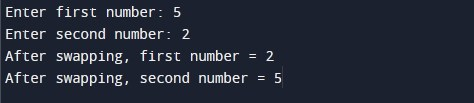# C Program to Swap two numbers using pointers

In this tutorial you will learn about the C Program to Swap two numbers using pointers and its application with practical example.

In this tutorial, we will learn to create a program to swap two numbers using pointers in C programming language.

Prerequisites

Before starting with this tutorial we assume that you are best aware of the following C programming topics:

• C Data Types
• C Variables
• C Input Output
• C Operators
• C Pointer

## Program to swap two numbers using pointers

In this program we will swap two integer numbers using pointers. We have first declared and initialized the required variables. Next, we would prompt user to input two integer numbers. Later in the program we will swap numbers using pointers. Finally, we will display number values after swapping of numbers.

Output:-In the above program, we have first declared and initialized a set variables required in the program.

• num1 = it holds the first integer number value
• num2 = it holds the second integer number value
• *a = pointer variable
• *b = pointer variable
• temp = it is used to hold temporary value

In the next statement user will be prompted to enter the two integer number values which will be assigned to variable ‘num1’ and ‘num2’ respectively. Next, we will swap two integer numbers using pointer variable *a and *b variable. Finally, we will display number values after swapping of numbers.

In this tutorial we have learn about the C Program to Swap two numbers using pointers and its application with practical example. I hope you will like this tutorial.